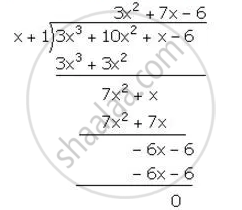Share

# Using the Remainder Theorem, Factorise the Expression 3x3 + 10x2 + X – 6. Hence, Solve the Equation 3x3 + 10x2 + X – 6 = 0. Hence, Solve the Equation 3x3 + 10x2 + X – 6=0 - Mathematics

Course

#### Question

Using the Remainder Theorem, factorise the expression 3x3 + 10x2 + x – 6. Hence, solve the equation 3x3 + 10x2 + x – 6 = 0.

#### Solution

"Left f "(x)=3x^3+10x^2+x-6

for x =-1,

f(x)=f(-1)=3(-1)^3+10(-1)^2+(-1)-6=-3+10-1-6=0

"Hence,(x+1) is a  factor of f(x)" .∴ 3x^3+10x^2+x-6=(x+1)(3x^2+7x-6)

=(x+1)(3x^2+9x-2x-6)

= (x-1)[3x(x-3)-2(x+3)]

=(x+1)(x+3)(3x-2)

now, 3x^3+10x^2+x-6=0

(x+1)(x+3)(3x-2)=0

x=-1, -3, 2/3

Is there an error in this question or solution?

#### APPEARS IN

Selina Solution for Concise Mathematics for Class 10 ICSE (2020 (Latest))
Chapter 8: Remainder and Factor Theorems
Exercise 8(B) | Q: 3 | Page no. 112
Solution Using the Remainder Theorem, Factorise the Expression 3x3 + 10x2 + X – 6. Hence, Solve the Equation 3x3 + 10x2 + X – 6 = 0. Hence, Solve the Equation 3x3 + 10x2 + X – 6=0 Concept: Factorising a Polynomial Completely After Obtaining One Factor by Factor Theorem.
S